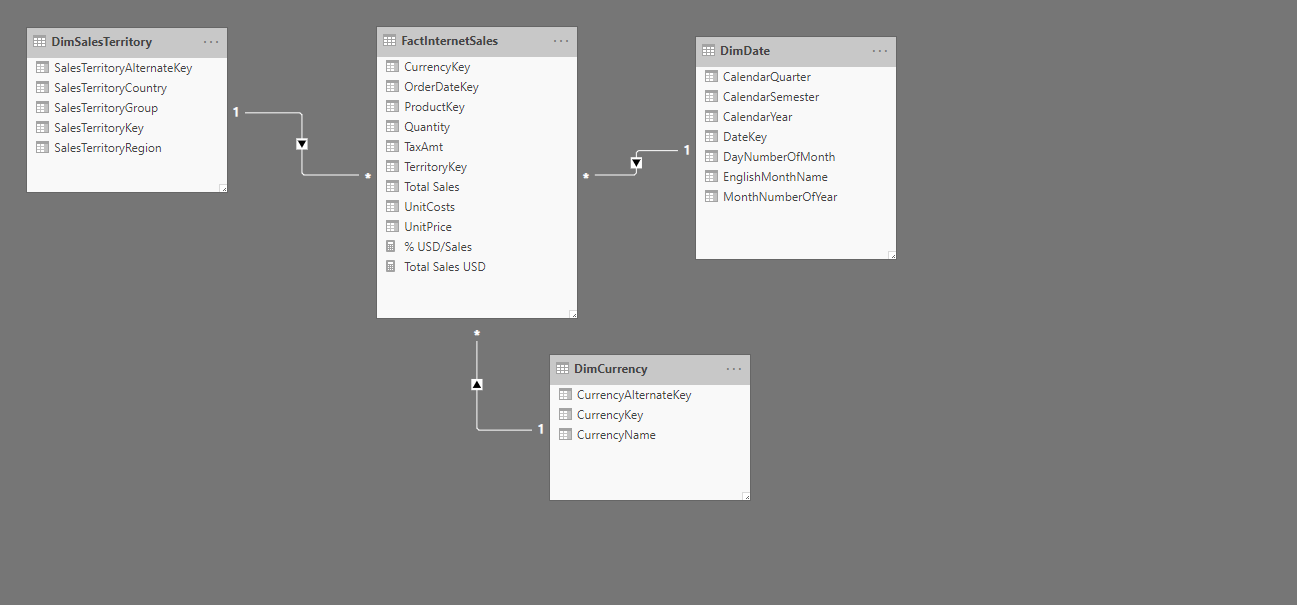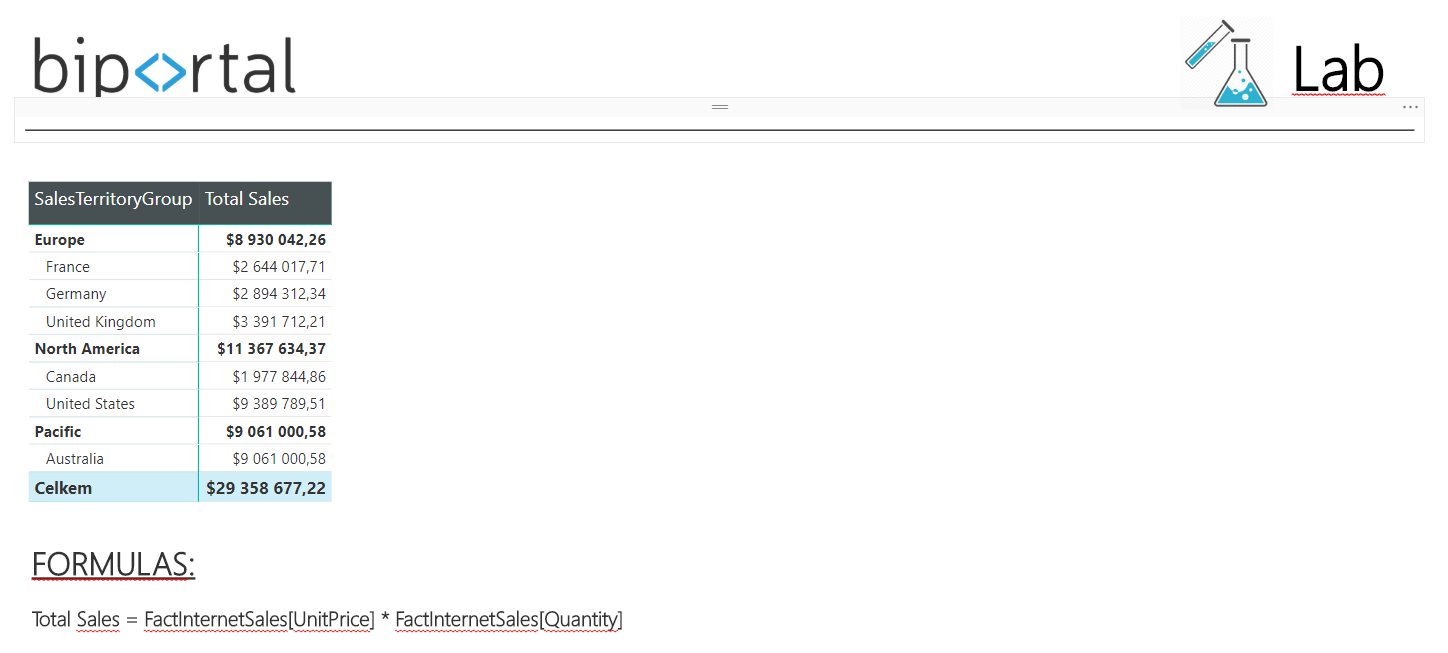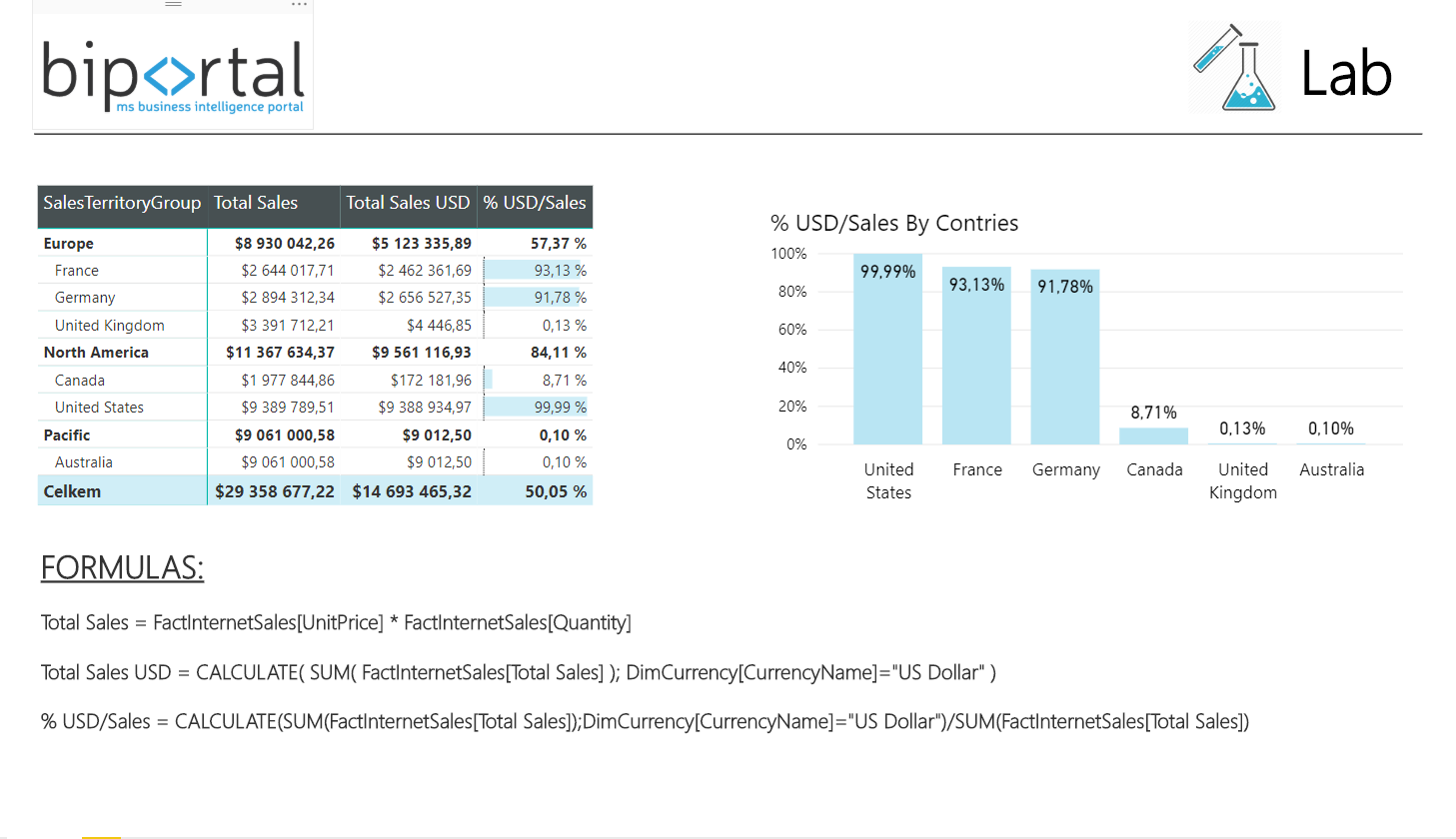• 21. 3. 2020
• Ing. Jan Zedníček - Data & Finance
• 0

CALCULATE is an important function for a large number of calculations and for those of you who actively do DAX formulas in Power BI or PowerPivot. Understanding of how the function works and how it is used does not look simple at first glance. And that is why this article is here.

Syntax function: CALCULATE( <expression>, <filter 1>, <filter 2>… )• Expression: First parameter is expression. In most of the cases it is some agreggate DAX function of type SUM, MIN, MAX, COUNTROWS or its colleague X function (e.g. SUMX).
• Filter 1 – Filter n: We then enter filter type parameter. There is plenty of filters available and it is also possible to apply some sort of AND/OR logic. Filter can be set in many ways:
• As a simple condition of type Product-“auto”…
• or as a next function. Most of table function FILTER which works as a condition – it limits values

Function example:

Blue cars sales = CALCULATE ( SUM ( Sales[Amount] ), Product[ProductColor] = “blue” )

Caution: Expression entered into first parameter must be evaluated in a way so that the result will always be 1 value. Result of the function is not a table but a value. This is why we mostly use aggregate functions.

## CALCULATE Function on Example in Power BI

Lets demonstrate the function on some examples in Power BI desktop environment. I will work with four tables containing sales (FactInternetSales), Territories, Currencies (DimCurrency) and Calendar (DimDate) as seen in the model.Step 1) I will start simple. Simple Power BI report with table will be prepared. New columns will be then added into it. I will display Sales by territories for now:Step 2)  I am interested in total sales realized in USD in the next column which will be displayed. And the last column will be a ratio between both previous columns and it will show what is the ration of sales in USD compared to all sales in %. Finally, DAX function CALCULATE comes into play:

Formula Total Sales USD:

`Total Sales USD :=`
` CALCULATE (`
`     SUM ( FactInternetSales[Total Sales] ),`
` DimCurrency[CurrencyName] = "US Dollar"`
` )`

Formula % USD/Sales:

`% USD/Sales =`
`CALCULATE (`
`    SUM ( FactInternetSales[Total Sales] ),`
`    DimCurrency[CurrencyName] = "US Dollar"`
`)`
`    / SUM ( FactInternetSales[Total Sales] )`

Result (as below) is fine and we see everything needed – total sales, sales in USD and its ratio. The result can be visualized by some graph and it is done.5/5 - (1 vote)### Ing. Jan Zedníček - Data & Finance

My name is Jan Zedníček and I work as a freelancer. I have been working as a freelancer for many companies for more than 10 years.
I used to work as a financial controller, analyst and manager at many different companies in field of banking and manufacturing. When I am not at work, I like playing volleyball, chess, doing a workout in the gym.